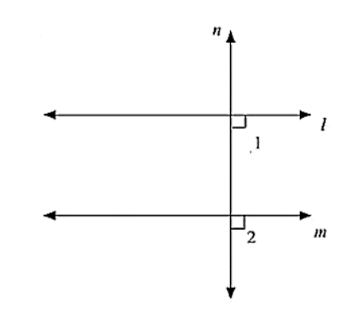# If two straight lines are perpendicular to the same line, prove that they are parallel to each other.

Question:

If two straight lines are perpendicular to the same line, prove that they are parallel to each other.Solution:

Given m perpendicular t and l perpendicular to t

∠1 = ∠2 = 90°

Since, I and m are two lines and it is transversal and the corresponding angles are equal

L ∥ M

Hence proved